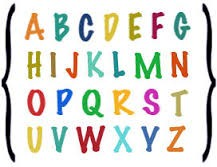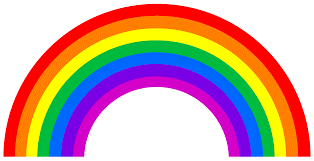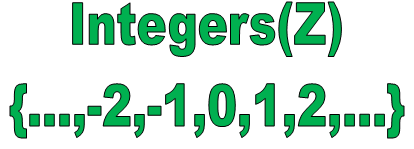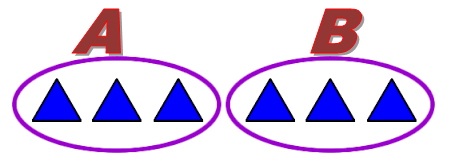×#### Thank you for registering.

One of our academic counsellors will contact you within 1 working day.

Click to Chat

1800-1023-196

+91-120-4616500

CART 0

• 0

MY CART (5)

Use Coupon: CART20 and get 20% off on all online Study Material

ITEM
DETAILS
MRP
DISCOUNT
FINAL PRICE
Total Price: Rs.

There are no items in this cart.
Continue Shopping```Finite and Infinite Sets

Table of Content

Definition of Finite Set

Cardinality of a Finite Set

Properties of Finite Sets

Examples of Finite Sets

Is Empty Set a Finite Set?

Definition of Infinite Sets

Cardinality of Infinite Sets

Properties of Infinite Sets

Examples of Infinite Sets

What is the meaning of Equal Sets in Math?

What is the difference between Finite and Infinite Sets?

How to determine if a Set is Finite or Infinite?

What is Finite or Infinite?

Graphical Representation of Finite and Infinite Sets

Definition of Finite Set

As the name represents, the finite set is a set having finite or countable number of elements.

ExampleIt is a set of all English alphabets.

As we can count the number of elements here , so it a finite set.

Cardinality of a Finite Set

The cardinality of a finite set is n(A)= a, where, a represents the number of elements of set A.

As in the above picture , the cardinality of this set is 26, as the number of elements are 26.

So, n (A) = 26.

This shows that if you can list all the elements of a set and write them in the curly braces or you can say in Roster form are called the finite sets.

Sometimes it may possible that the number of elements is very big but somewhere it is countable or it has starting and end point then it is a non empty finite set.Here we denote the number of elements with n(A) and if n(A) is a natural number then you can say that it is a finite set.

Properties of Finite Sets

The subset of a finite set is always finite.

The union of two finite sets is finite.

The power set of a finite set is finite.

Let’s see with example

A = {1, 2, 3, 4}

B = {2, 4, 6, 8}

C  = {2, 3}

Here, all A,B and C are the finite sets as there number of elements are limited and countable.

C⊂A,i.e., C is the subset of A , as all the elements of set C are present in set A.So the subset of a finite set is always finite.

A B is {1, 2, 3, 4, 6, 8}, so the union of two finite sets is also finite.

The number of elements of a power set of a set is 2n, so the number of elements of the power set of set A is 25 = 32, as the number of elements of set A is 5.This shows that the power set of a finite set is finite.

Example

Z = { a set of number of people live in europe}

In this example, it is difficult to count the number of people live in Europe, but it is somewhere a natural number. So it is a non empty finite set.

The finite set can be represented in sequence,

N is a set of natural numbers less then n.So the cardinality of set N is n.

N = {1, 2, 3,…, n}

Y = y1, y2,…, n

Y = {y:y1∈N,y1≤i≤n}, where i is the integers between 1 and n.

Examples of Finite SetsSet of all colors of rainbow.

R = {Violet, Indigo, Blue, green, yellow, orange, red}

n(R) = 7

Set of all natural numbers between 25 and 100.

N= {25, 26, 27,…, 100}

n(N) =76

Set of all days in a week.

D = {Monday, Tuesday, Wednesday, Thursday, Friday, Saturday, Sunday}

n (D) =7

All the above are the examples of finite sets because the number of elements is countable in them. And their cardinality is a natural number.

Is empty Set a Finite Set?

To understand the answer of this question, first we need to understand the meaning of Empty set.

Empty Set – Empty set is a set having no element in it. It can be represented as { }, which shows that there is no element in a set.

The cardinality of an empty set is 0 (zero), i.e., the number of elements is zero.

A= { } or ∅ (phi)

n (A) = 0

The finite set is a set with a countable number of elements and as the empty set has zero element in it, so it is definite number of element.

The empty set is a finite set with a cardinality of zero.

Definition of Infinite Sets

A set which is not a finite set is called an Infinite Set. Or if you cannot count the number of elements of a particular set then it is said to be an infinite set.

As we represent a finite set in roster form, we cannot represent an infinite set in roster form easily as its elements are not limited so we use the three dots (ellipses) to represent the infinity of a set.

ExampleAs in the above example,

N is a set of all natural numbers starting from zero. Its number of elements is not countable so we use the three dots to represent its infinity.

Z is a set of all integers, as its elements are also uncountable so we use the three dots both the sides for the infinity of negative and positive integers.

It is important to note that the sets must have some well defined structure or pattern then only we can write it in roster form, so it is not possible to write all the infinite sets in roster form.

As we cannot write the set of real numbers in roster form as there is no proper structure of these numbers.

Cardinality of Infinite Sets

Cardinality of a set is n (A) = x, where x is the number of elements of a set A.

As the number of elements in an infinite set is unlimited, so the cardinality of an infinite set is n (A) = ∞, i.e., infinite.

Properties of Infinite Sets

The union of two infinite sets is infinite

The power set of an infinite set is infinite

The super set of an infinite set is also infinite

As the number of elements of an infinite set is unlimited so its power set and supersets also need to be infinite.

Examples of Infinite Sets

A set of all whole numbers.

W= {1, 2, 3, 4,…}

A set of all points on a line.

A set of all triangles.

What is the meaning of Equal Sets in Math?

In mathematics, we said a number equal to other if they are exactly same. Similarly in sets, we said two sets to be equal if there all the elements are same. The order of elements and the repetition of elements do not have any relevance.Here, Set A and Set B are equal sets as there elements are exactly same.and there number of elements is also same.

Example

A = {5, 6, 7, 8}

B = {6, 8, 5, 7}

C = {5, 5, 6, 6, 7, 7, 8, 8}

Here all the three sets, set A, set B and set C are equal, as there elements are same irrelevance of order and the repetition.

What is the difference between Finite and Infinite Sets?

The difference between finite and infinite sets is as follows:

The sets could be equal only if there elements are same, so a set could be equal only if it is a finite set. And if a set is infinite, we cannot compare the elements of the sets.

No.

Points

Finite sets

Infinite sets

1

Definition

A set is a finite set if it is empty or a limited number of elements.

A set which is not a finite set is an infinite set.

2

Number of elements

Countable number of elements.

Uncountable number of elements.

3

Continuity

It starts and also stops.

It has no end either in the beginning or in last or could have both sides’ continuous.

4

Cardinality

n (A) = n, n is the number of elements.

n (A) =∞, infinite as the number of elements are uncountable.

5

Union

Union of two finite sets is finite.

Union of two infinite sets is infinite.

6

Power set

Power set of a finite set is finite.

Power set of an infinite set is infinite.

7

Roster form

Can be easily represented in roster form.

All sets cannot be shown in roster form so we use three dots to represent the infinity.

8

Example

A = {2, 4, 6, 8}

X = {2, 4, 6, 8,…}

A set of even numbers less than 9.

A set of all even numbers.

How to determine if a Set is Finite or Infinite?

As we know that a set is finite if it has a starting point and an ending point both, but a set is said to be infinite if it has no end from any side or both sides.

Points to determine a set as finite or infinite are:

If a set has a starting and end point both then it is finite but if it does not have a starting or end point then it is infinite set.

If a set has a limited number of elements then it is finite but if its number of elements is unlimited then it is infinite.

What is Finite or Infinite?

Let’s try to determine a set whether it is finite or infinite with their elements.

No.

Example

Finite or Infinite

Why?

1

A={5,10,15,20}

Finite

This set has both starting point and ending point and its number of elements are limited.

2

B= {5, 10, 15, 20,…}

Infinite

This set has a starting point but not an ending point. As the multiples of 5 could not be countable.

3

C= {…,-2,-1, 0, 1, 2,…}

Infinite

This set has no starting point and not even an end point, so its number of elements is uncountable.

4

D= {x:x W and 0<x<10}

Finite

This is the set of whole numbers between 0 and 10. So has limited number of elements.

5

E={x:x∈R and x-2=10}

Finite

This is a set of real numbers where the elements of this set are those where x-2=10.As the set of real numbers is infinite but here the equal sign make it finite.

6

F={x:x∈R and x+4>12}

Infinite

Here the elements of this set are x+4 is anything greater than 12. As the set of real numbers is infinite so there is no end point of this set.

Graphical Representation of Finite and Infinite SetsHere in the above picture,

H = {a, s, h, e, d}

T = {a, s, t, i, l}

HUT = {a, s, h, e, d, t, i, l}

H∩T = {a, s}

Both H and T are finite sets as they have limited number of elements.

n(H) = 5 and n(T) = 5

HUT and H∩T are also finite.

This shows that we can easily represent the finite sets through venn diagram .

The union of two finite sets is finite.

The intersection of two finite sets is also finite.

But it is difficult to represent an infinite set with venn diagram, as it has unlimited number of elements and it can not be bounded in a circle to represent.

Finite and Infinite Sets
```### Course Features

• 731 Video Lectures
• Revision Notes
• Previous Year Papers
• Mind Map
• Study Planner
• NCERT Solutions
• Discussion Forum
• Test paper with Video Solution